## The shape of a toy is given as f(x)=6(2x 4 - x 2 ). To make the toy beautiful 2 sticks which are perpendicular to each other were placed at a point (2, 3), above the toy.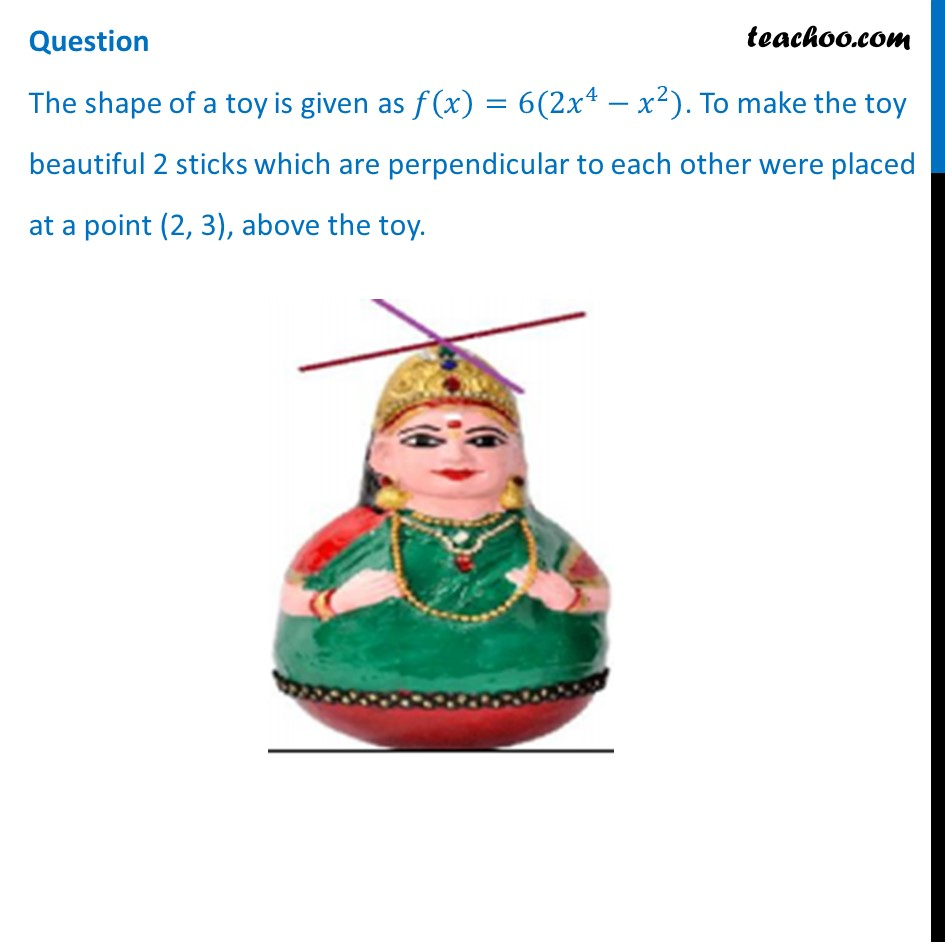## (d) None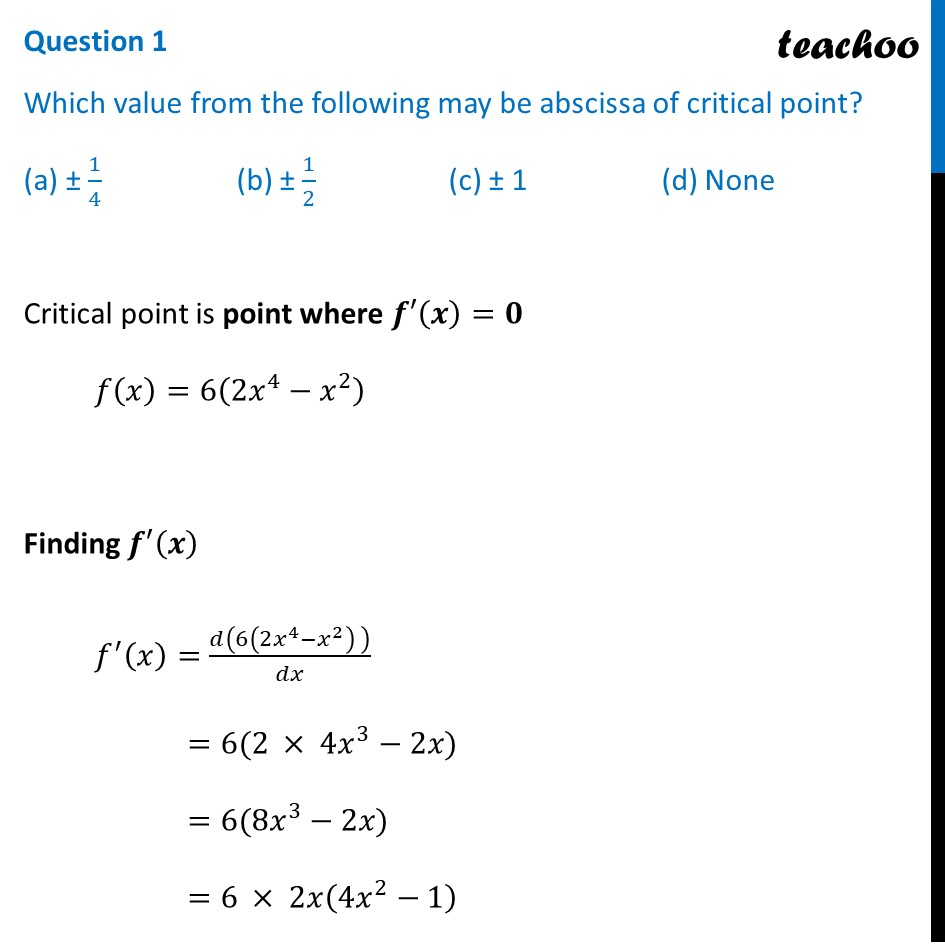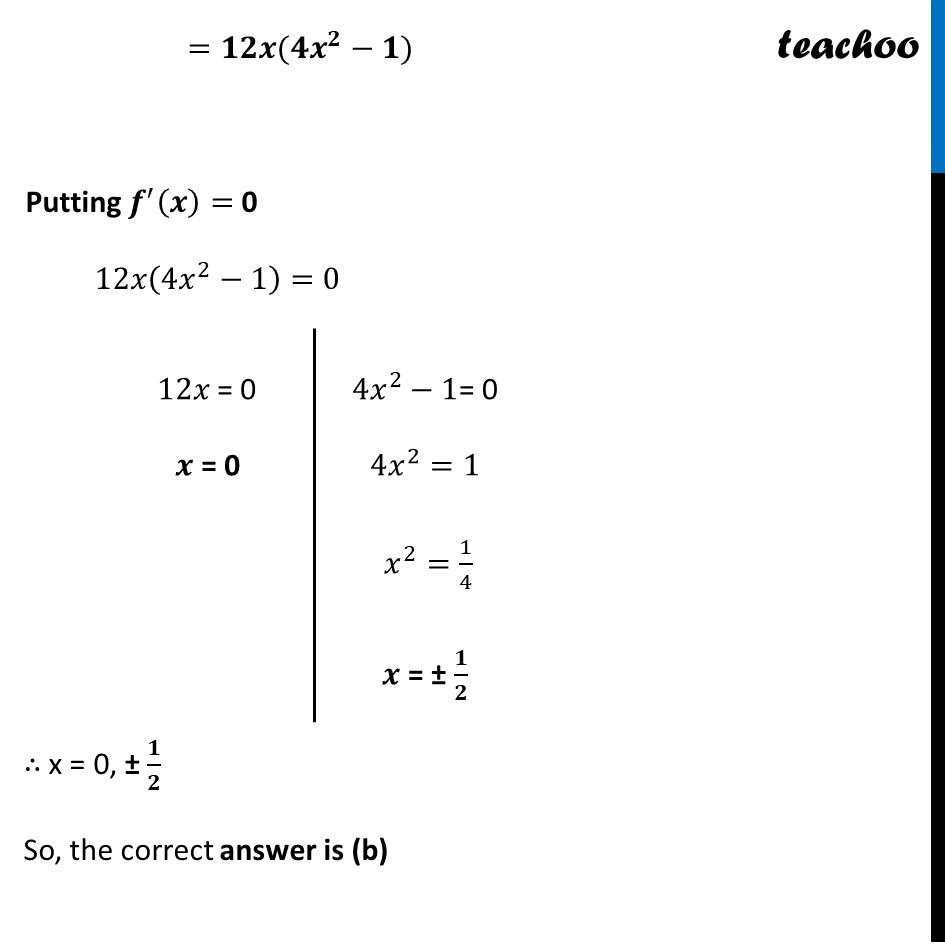## (d) (-1)/360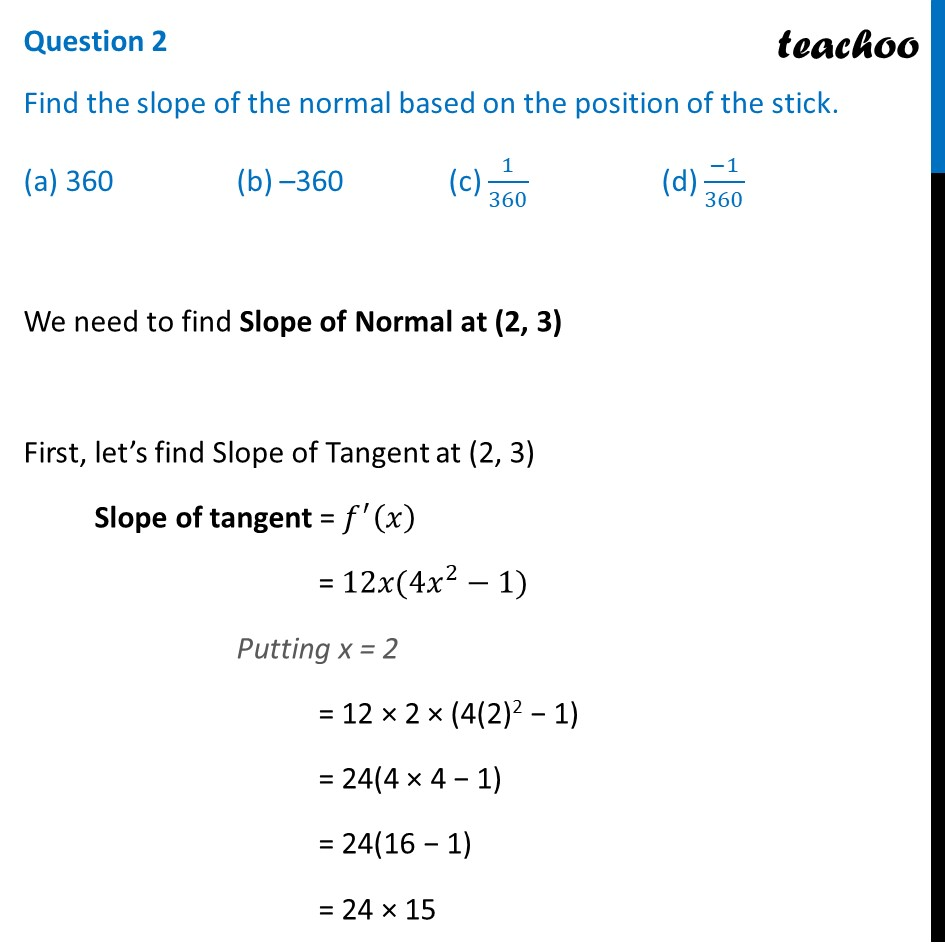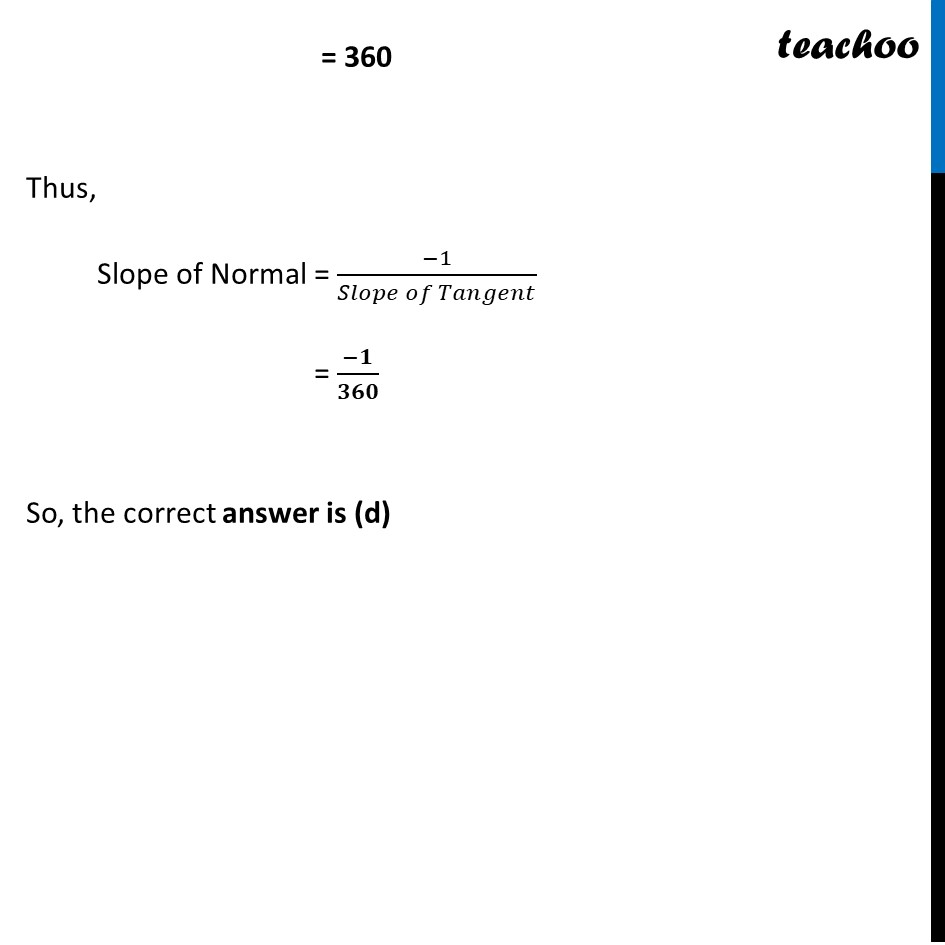## (d) none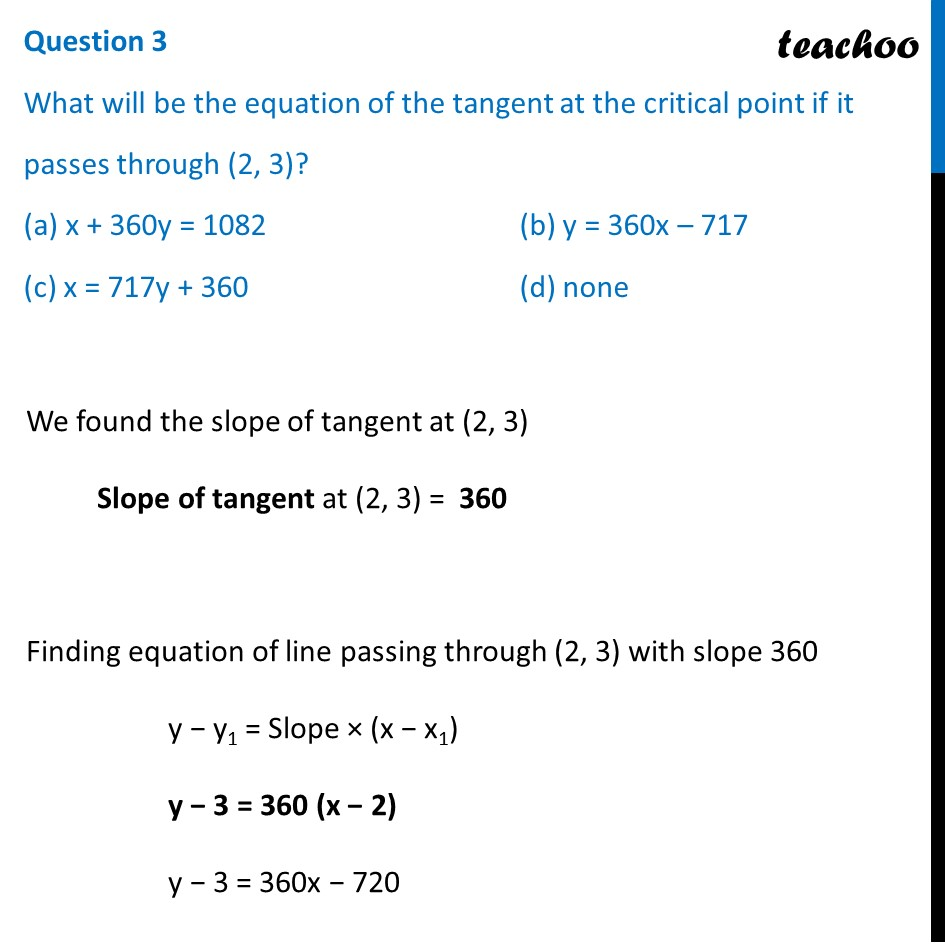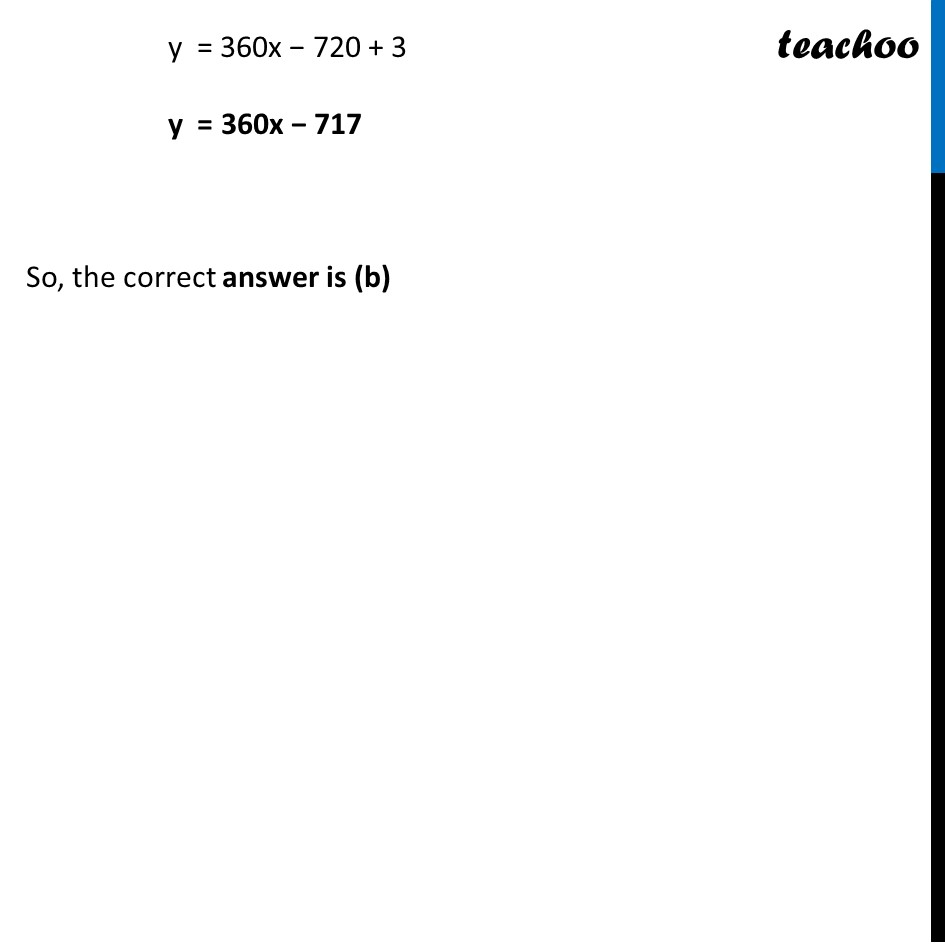## (d) 3312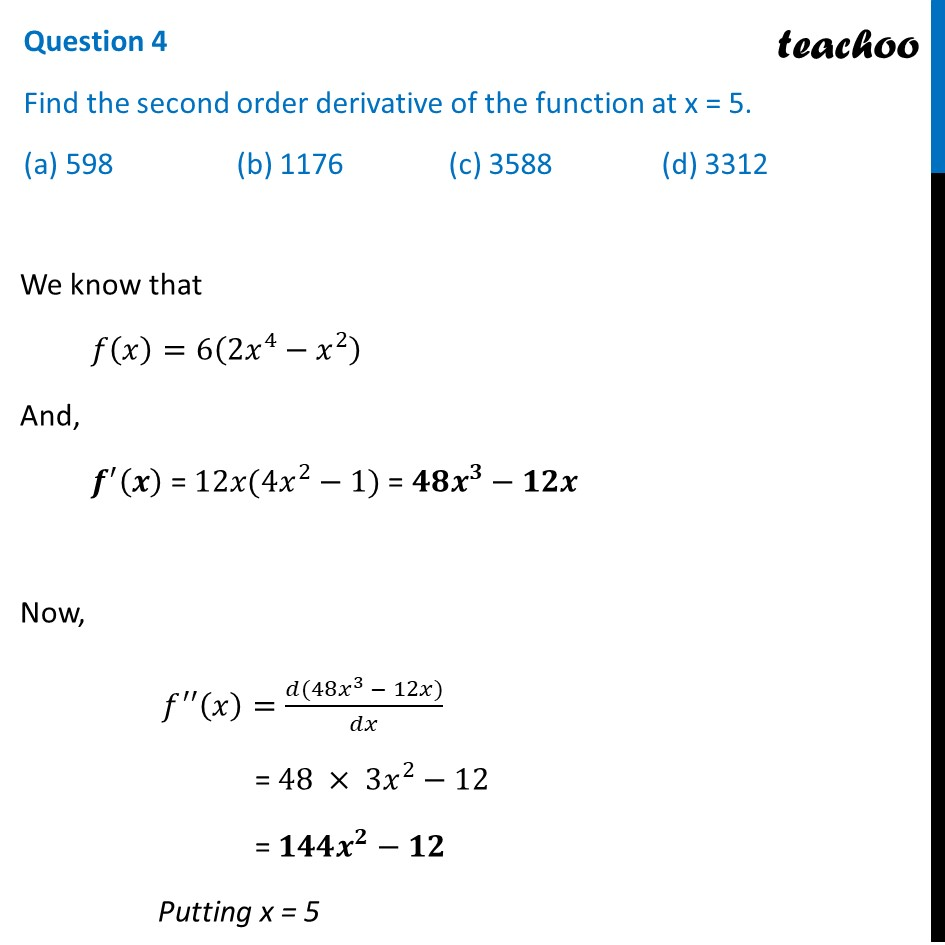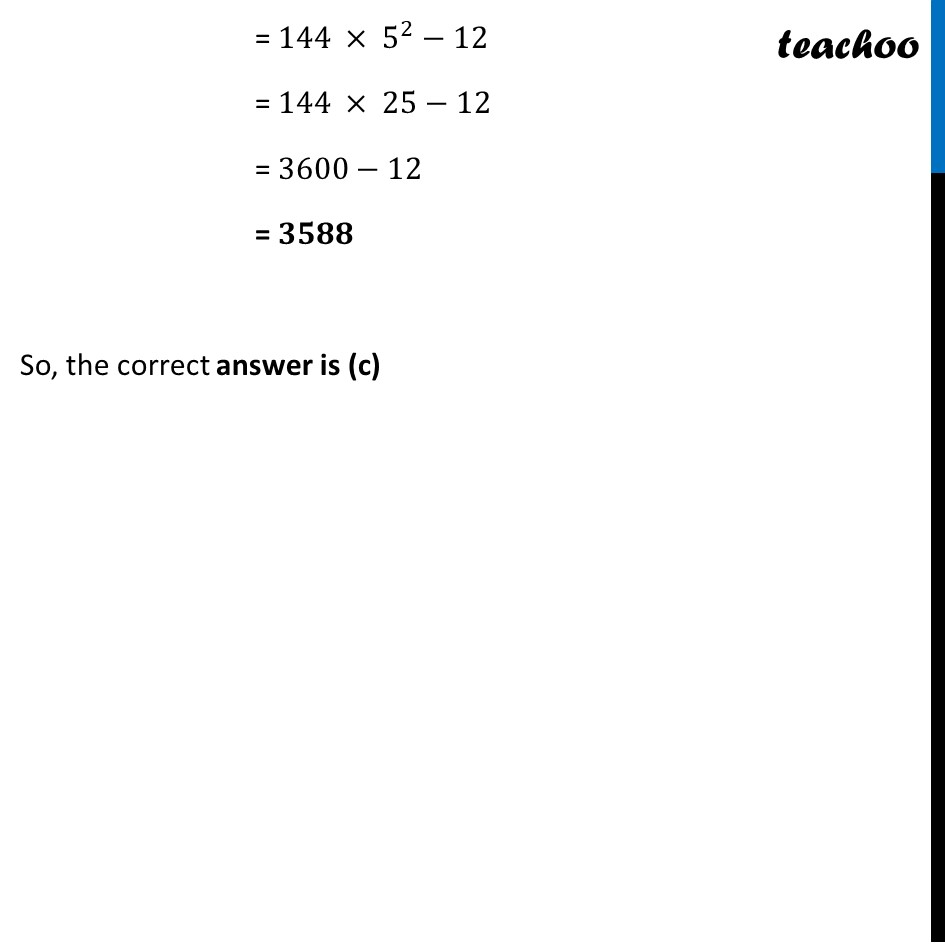## Question 5 At which of the following intervals will f(x) be increasing? (a) (-∞", "  (-1)/2) ∪ (1/2 ", " ∞) (b) (1/2,0) ∪ (1/2 ", " ∞) (c) (0", "  1/2) ∪ (1/2 ", " ∞) (d) (-∞", "  (-1)/2) ∪ (0", "  1/2)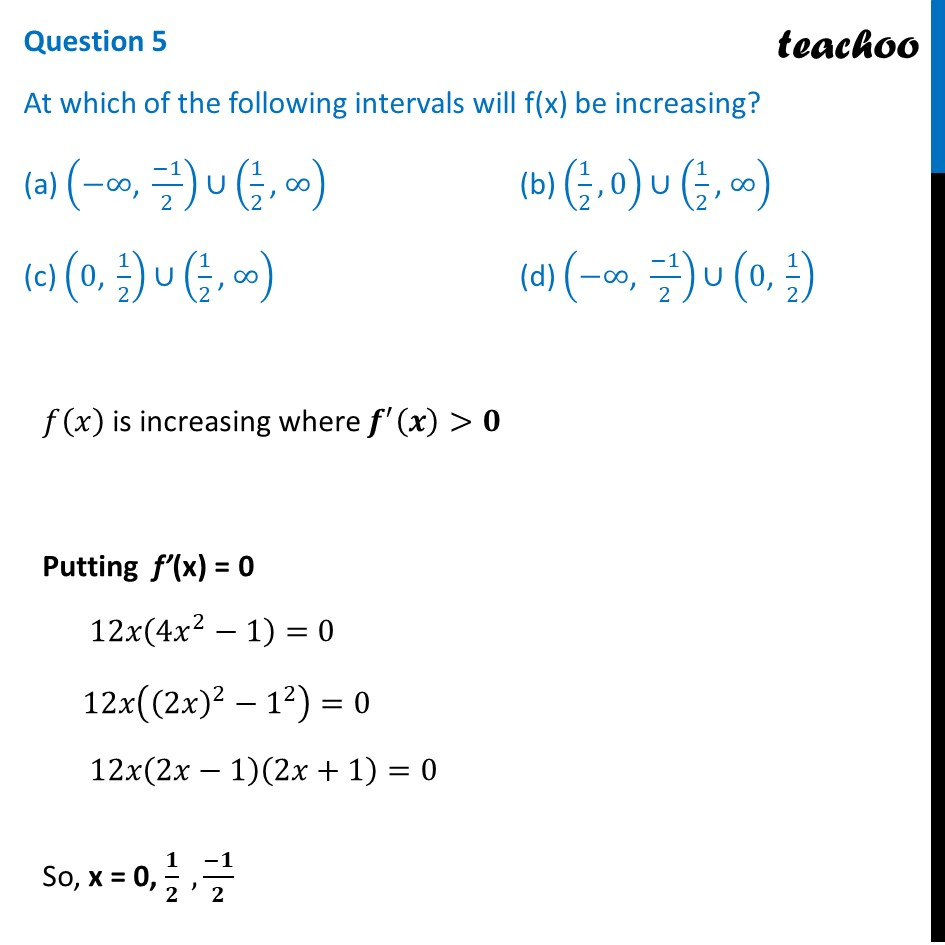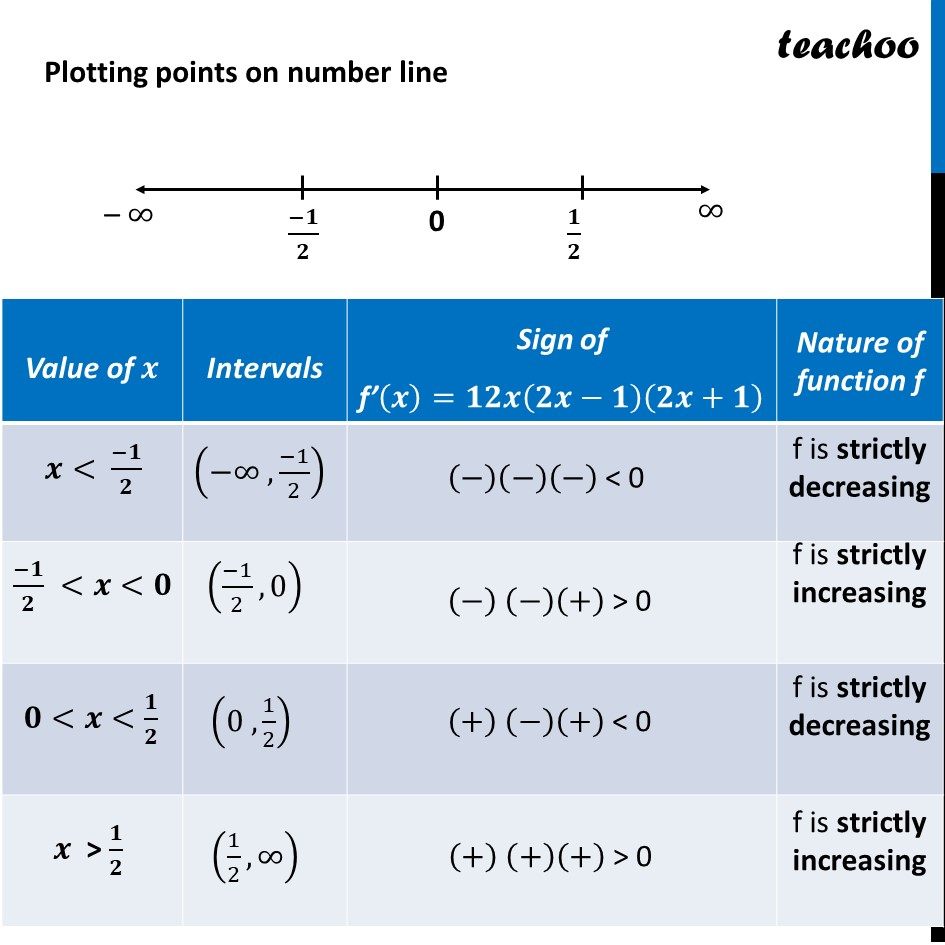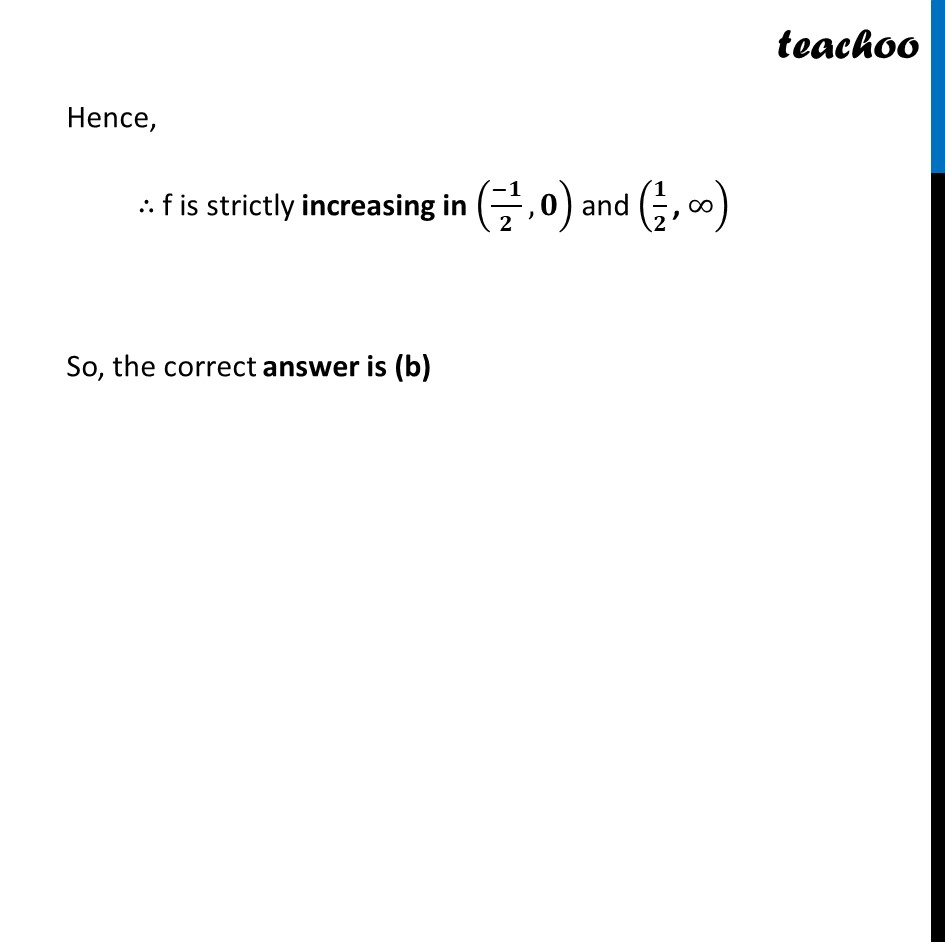1. Chapter 6 Class 12 Application of Derivatives (Term 1)
2. Serial order wise
3. Case Based Questions (MCQ)

Transcript

Question The shape of a toy is given as 𝑓(𝑥)=6(2𝑥^4−𝑥^2). To make the toy beautiful 2 sticks which are perpendicular to each other were placed at a point (2, 3), above the toy. Question 1 Which value from the following may be abscissa of critical point? (a) ± 1/4 (b) ± 1/2 (c) ± 1 (d) None Critical point is point where 𝒇′(𝒙)=𝟎 𝑓(𝑥)=6(2𝑥^4−𝑥^2 ) Finding 𝒇′(𝒙) 𝑓^′ (𝑥)=𝑑(6(2𝑥^4−𝑥^2 ) )/𝑑𝑥 =6(2 × 4𝑥^3−2𝑥) =6(8𝑥^3−2𝑥) =6 × 2𝑥(4𝑥^2−1) =𝟏𝟐𝒙(𝟒𝒙^𝟐−𝟏) Putting 𝒇^′ (𝒙)= 0 12𝑥(4𝑥^2−1)=0 12𝑥 = 0 𝒙 = 0 4𝑥^2−1= 0 4𝑥^2=1 𝑥^2=1/4 𝒙 = ± 𝟏/𝟐 ∴ x = 0, ± 𝟏/𝟐 So, the correct answer is (b) Question 2 Find the slope of the normal based on the position of the stick. (a) 360 (b) –360 (c) 1/360 (d) (−1)/360 We need to find Slope of Normal at (2, 3) First, let’s find Slope of Tangent at (2, 3) Slope of tangent = 𝑓^′ (𝑥) = 12𝑥(4𝑥^2−1) Putting x = 2 = 12 × 2 × (4(2)2 − 1) = 24(4 × 4 − 1) = 24(16 − 1) = 24 × 15 = 360 Thus, Slope of Normal = (−1)/(𝑆𝑙𝑜𝑝𝑒 𝑜𝑓 𝑇𝑎𝑛𝑔𝑒𝑛𝑡) = (−𝟏)/𝟑𝟔𝟎 So, the correct answer is (d) Question 3 What will be the equation of the tangent at the critical point if it passes through (2, 3)? (a) x + 360y = 1082 (b) y = 360x – 717 (c) x = 717y + 360 (d) none We found the slope of tangent at (2, 3) Slope of tangent at (2, 3) = 360 Finding equation of line passing through (2, 3) with slope 360 y − y1 = Slope × (x − x1) y − 3 = 360 (x − 2) y − 3 = 360x − 720 y = 360x − 720 + 3 y = 360x − 717 So, the correct answer is (b) Question 4 Find the second order derivative of the function at x = 5. (a) 598 (b) 1176 (c) 3588 (d) 3312 We know that 𝑓(𝑥)=6(2𝑥^4−𝑥^2 ) And, 𝒇^′ (𝒙) = 12𝑥(4𝑥^2−1) = 𝟒𝟖𝒙^𝟑−𝟏𝟐𝒙 Now, 𝑓^′′ (𝑥)=(𝑑(48𝑥^3 − 12𝑥))/𝑑𝑥 = 48 × 3𝑥^2−12 = 𝟏𝟒𝟒𝒙^𝟐−𝟏𝟐 Putting x = 5 = 144 × 5^2−12 = 144 × 25−12 = 3600−12 = 𝟑𝟓𝟖𝟖 So, the correct answer is (c) Question 5 At which of the following intervals will f(x) be increasing? (a) (−∞", " (−1)/2) ∪ (1/2 ", " ∞) (b) (1/2,0) ∪ (1/2 ", " ∞) (c) (0", " 1/2) ∪ (1/2 ", " ∞) (d) (−∞", " (−1)/2) ∪ (0", " 1/2) 𝑓(𝑥) is increasing where 𝒇^′ (𝒙)>𝟎 Putting f’(x) = 0 12𝑥(4𝑥^2−1)=0 12𝑥((2𝑥)^2−1^2 )=0 12𝑥(2𝑥−1)(2𝑥+1)=0 So, x = 0, 𝟏/𝟐 , (−𝟏)/𝟐 Hence, ∴ f is strictly increasing in ((−𝟏)/𝟐,𝟎) and (𝟏/𝟐 ", " ∞) So, the correct answer is (b)

Case Based Questions (MCQ)